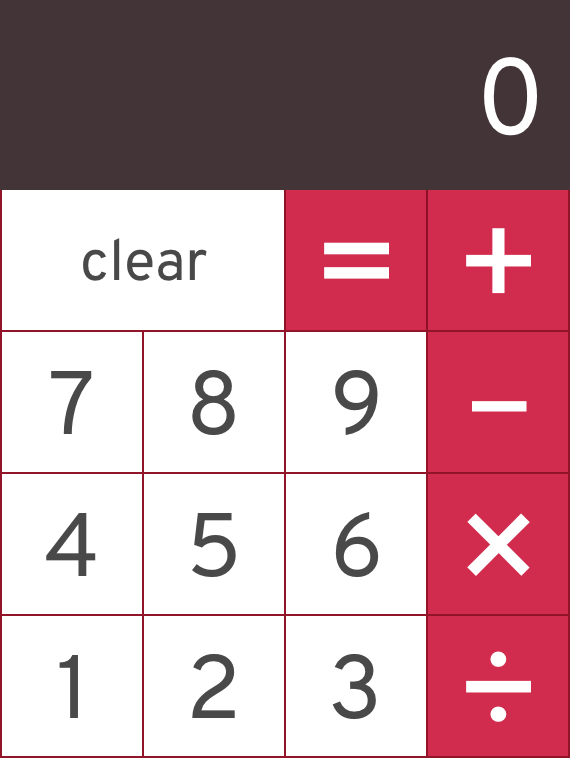Note: This document is a work in progress. You can help improve it.

## Calculator Fun

Practice is key to gaining the mileage needed conquer programming. A very common practice problem is to recreate a working calculator. A basic calculator is a good exercise in using state, effects and components to create a powerful and robust UI.

### Objectives

• practice using components
• practice implementing a UI wireframe

### Requirements

Create a simple calculator that allows the user to add, multiple, subtract and divide two numbers. You implement this ui:#### Explorer Mode

• Implement the Above UI using HTML and CSS inside a react app
• As a user, I should be able to add two numbers
• As a user, I should be able to add subtract numbers
• As a user, I should be able to add multiple numbers
• As a user, I should be able to add divide numbers
• The clear button should reset the current calculation

• Be able to chain together results. As in, the user should be able to input `2 + 3 - 4 + 5` and get an answer